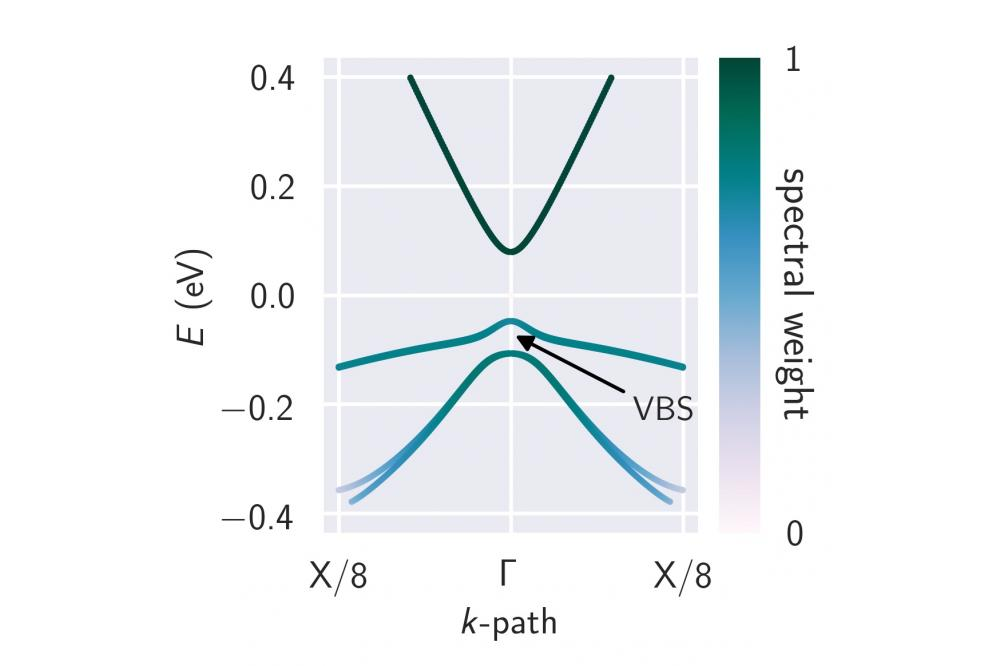# DFT Based Modeling of the Optical Properties of Dilute BismidesDFT based modeling of the optical properties of dilute bismides

Figure 1: Band structure of InAs0.92Bi0.08 in the vicinity of the Brillouin zone center (Γ-point). Shown are the lowest conduction band (energetically highest band shown), the heavy-hole band (band in the middle) and the light-hole band (energetically lowest band shown). The gap between the heavy-hole and the light-hole band at the Γ-point corresponds to the observed valence band splitting (VBS).

## Introduction

For the modern communication and information technology semiconductors with improved optoelectronic properties are highly desirable. For instance, semiconductor lasers based on the InP material system, which are used in telecommunication, suffer from intrinsic loss mechanisms and therefore need to be cooled in order to maintain stable laser operation conditions. One of these loss mechanisms is the so called Auger process which refers to a radiation-free recombination of charge carriers. Novel semiconductor materials such as Ga(AsBi), Ga(AsBiN), (GaIn)(AsBi) and alike, which are commonly referred to as dilute bismides, are promising candidates for more efficient semiconductor lasers. We develop and use an ab initio based approach to predict the optoelectronic properties and losses for these materials. By making use of this approach, the efficiency of the development process for new semiconductor materials can be enhanced.

## Methods

In order to compute the optical properties and intrinsic losses of the materials in question, certain information on their electronic properties is required. Density functional theory is an efficient ab initio method, i.e. (almost) no input other than the crystal structure of the material is required, to obtain the needed information. Subsequently, the optical properties and losses are calculated making use of a many-body quantum theory that has proven to yield reliable results for many established semiconductor materials. Since we are interested in materials with dilute, i.e. very small, amounts of bismuth, we require large supercells in our density functional theory calculations. These calculations can easily become very demanding on computational power and memory which is the reason why we rely on a high-performance cluster. The subsequent calculations of the optical properties and losses based on the results obtained on the cluster can be performed on workstations.

## Results

We used density functional theory to calculate band structures such as the one shown in Figure 1 for InAs0.92Bi0.08. Thereby, in some of our calculations we identified a splitting of the two topmost valence bands. This splitting is due to a local loss of the crystal symmetry caused by the incorporation of Bi atoms into the symmetric InAs crystal structure. In order to have a more convenient access to the band structure, we modified an existing empirical approach by including the observed valence band splitting. For this, several density functional theory calculations were carried out which allowed for a parametrization of the valence band splitting for In(AsBi) and Ga(AsBi).

Moreover, we compared the optical properties and losses using input directly from density functional theory and input from the empirical approach for the optical calculations. Thereby, the limiting cases in which the empirical approach is valid were identified. Overall, we find that for low Bi content, where the valence band splitting is small, using the empirical model suffices to calculate the optical properties.

However, for the calculation of the Auger losses there is a huge discrepancy for the loss coefficients also for low Bi content and input from density functional theory calculations needs to be used.

We find that for InAs the calculated loss coefficient is in agreement with reported values from the literature. For In(AsBi) the loss coefficient increases nearly exponentially with rising Bi content.

## Outlook

Although the energy bands of In(AsBi) are such that one particular Auger process is suppressed, we find a loss coefficient that is by 2-3 orders of magnitude larger than for the (InGa)As material system, which emits in a similar spectral range. Therefore, our results for the loss coefficient of In(AsBi) point towards the need of investigating more complex compounds or even heterostructure alignments for identifying a system with low loss conditions.

## Last Update

• Last Update: 2021-08-19 13:57